## 15 Questions MCQ Test Mathematical Olympiad Class 8 | Olympiad Test: Achieves Section-1

Description
Attempt Olympiad Test: Achieves Section-1 | 15 questions in 30 minutes | Mock test for Class 8 preparation | Free important questions MCQ to study Mathematical Olympiad Class 8 for Class 8 Exam | Download free PDF with solutions
QUESTION: 1

### The area of the base of a cone is 180 cm2.If the height of the cone is 8 cm what is its volume?

Solution:

Area of base = 180 cm2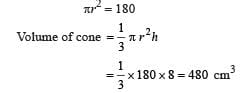QUESTION: 2

### The difference between the S.I. and C.I. on ₹ 2500 for 2 years at 20% when the compound interest is payable annually is

Solution: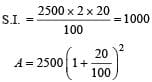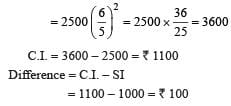QUESTION: 3

### Umesh tossed a coin three times. What is the probability that Umesh gets more heads than tails?

Solution:

Probability of getting 2 or 3 heads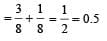QUESTION: 4

If 28 men can do a piece of work in 65 days. How many men will do it in 35 days?

Solution: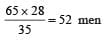QUESTION: 5

In 10 days earth picks up  2.6 × 108 kg of dust from the atmosphere. How much dusk will it pick up in 45 days?

Solution: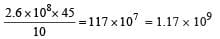QUESTION: 6

One of the factor of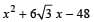is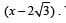. What is the other factor ?

Solution: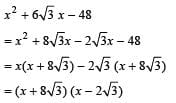QUESTION: 7

Two octagonal perfect dice with number 1 – 8 are thrown together. What is the probability that both the numbers are even ?

Solution:

Total no. of outcomes = 8 × 8 = 64
Both numbers are even,
{(2, 2) (2, 6) (2, 8) (2, 4) (4, 2) (4, 4), (4, 6) (4, 8), (6, 2), (6, 4) (6, 6), (6, 8) (8, 2) (8, 4) (8, 6), (8, 8)}.
Required probability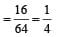QUESTION: 8

In what time will ₹ 64,000 amount to ₹ 68921 at 5% per annum if interest payable half yearly?

Solution: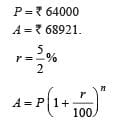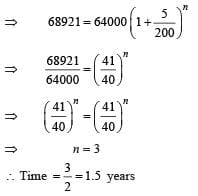QUESTION: 9

A mobile set is sold for ₹ 1498 and the seller gains 7% on it. What is its cost price?

Solution:

Let cost price be ₹ x.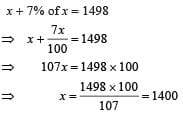QUESTION: 10

The sum of digits of a two-digit number is 9. If 9 is subtracted  from the number, its digits are interchanged.

Q. What is the half of that number?

Solution: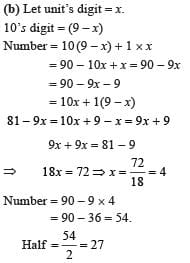QUESTION: 11

What is the value of x if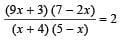Solution: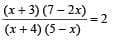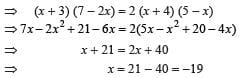QUESTION: 12

The curved surface area of the cylinder is 5940 cm2. If its height is 30 cm, what is the diameter of the base?

Solution:

Curved surface area = 5940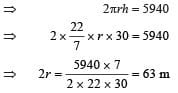QUESTION: 13

A cuboid vessel is 20 cm long and 12 cm wide. How high must it be to bold 3 liters of water?

Solution:

lbh = 3000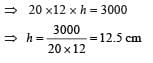QUESTION: 14

What is the value of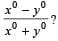Solution: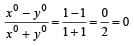QUESTION: 15

The square root of 0.0004 is

Solution: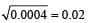Use Code STAYHOME200 and get INR 200 additional OFF Use Coupon Code